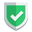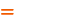我们相信：世界是美好的，你是我也是。平行空间的世界里面，不同版本的生活也在继续...

`neo4j`图数据库中，是由节点和关系所组成的。这些信息又是由节点标签、关系类型、属性等信息所组成的，并且这些最基本的部分在`neo4j`中，被归类于`schema`。在`neo4j browser`左侧中是被特殊列出的信息，在前些篇的文章中，苏南大叔对这些左侧列出的信息有所说明。本文是从代码角度来总结说明这些信息的，仅供参考。

## 直观观察一个图

``call db.schema.visualization``

## 获得所有节点标签

``````match (n) return labels(n)
match (n) return distinct(labels(n))``````

``call db.labels``

## 获得所有关系类型

``match (n)-[r]->(m) return distinct type(r)``

``call db.relationshipTypes``

## 获得所有节点属性

``match (n) unwind keys(n) as allkeys return distinct allkeys``

``````match (n) return distinct keys(n)
match (n:Person) return distinct keys(n)``````
`keys`是属性的键，`properties`是属性，是键+值，`unwind keys(n)`作用是把`key`的数组给打散开。
``````match (n) return distinct properties(n)
match (n)-[r]->(m) return distinct properties(n)
match (n)-[r]->(m) return distinct properties(m)``````

## 获得所有关系属性

``match (n)-[r]->(m) return properties(r)``

``match (n)-[r]->(m) return distinct keys(r)``

``match (n)-[r]->(m) unwind keys(r) as allkeys return distinct allkeys``

## 综述如果本文对您有帮助，或者节约了您的时间，欢迎打赏瓶饮料，建立下友谊关系。本博客不欢迎：各种镜像采集行为。请尊重原创文章内容，转载请保留作者链接。【福利】 腾讯云最新爆款活动！1核2G云服务器首年50元！【源码】本文代码片段及相关软件，请点此获取更多信息【绝密】秘籍文章入口，仅传授于有缘之人neo4j    cypher